# Spring Pendulum

A point mass suspended from a mass less spring or placed on a frictionless horizontal plane attached with spring (fig.) constitutes a linear harmonic spring pendulum

Time period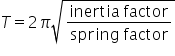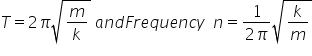Important Points

(i) Time period of a spring pendulum depends on the mass suspended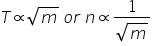i.e. greater the mass greater will be the inertia and so lesser will be the frequency of oscillation and greater will be the time period.

(ii) The time period depends on the force constant k of the spring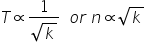(iii) Time of a spring pendulum is independent of acceleration due to gravity. That is why a clock based on spring pendulum will keep proper time every where on a hill or moon or in a satellite and time period of a spring pendulum will not change inside a liquid if damping effects are neglected.

(iv) If the spring has a mass M and mass m is suspended from it, effective mass is given by(v) If two masses of mass m1 and m2 are connected by a spring and made to oscillate on horizontal surface, the reduced mass mr is given by(vi) If a spring pendulum, oscillating in a vertical plane is made to oscillate on a horizontal surface, (or on inclined plane) time period will remain unchanged. However, equilibrium position for a spring in a horizontal plain is the position of natural length of spring as weight is balanced by reaction. While in case of vertical motion equilibrium position will be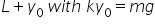(vii) If the stretch in a vertically loaded spring is  then for equilibrium of mass m,Time period does not depends on ‘g’ because along with g, yo will also change in such a way that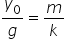remains constant

(viii) Series combination : If n springs of different force constant are connected in series having force constant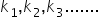respectively then(ix) Parallel combination : If the springs are connected in parallel then(x) If the spring of force constant k is divided in to n equal parts then spring constant of each part will become nk and if these n parts connected in parallel thenThat means if the length of spring is halved then its force constant becomes double.

(xii) When a spring of length l is cut in two pieces of length l1 and l2 such that .

If the constant of a spring is k then

Spring constant of first part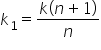Spring constant of second partRelated Keywords